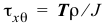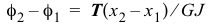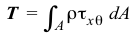# 5.1 Torsion of Circular Shafts

In the questions below, select the correct answer.

Name Email
1.

Torsional shear strain varies linearly across a homogeneous cross section.

2.

Torsional shear strain is a maximum at the outermost radius for a homogeneous and a nonhomogeneous cross section.

3.

Torsional shear stress is a maximum at the outermost radius for a homogeneous and a nonhomogeneous cross section.

4.

The formulacan be used to find the shear stress on a cross section of a tapered shaft.

5.

The formulacan be used to find the relative rotation of a segment of a tapered shaft.

6.

The formulacan be used to find the shear stress on a cross section of a shaft subjected to distributed torques.

7.

The formulacan be used to find the relative rotation of a segment of a shaft subjected to distributed torques.

8.

The equationcannot be used for nonlinear materials.

9.

The equationcan be used for a nonhomogeneous cross section.

10.

Internal torques jump by the value of the concentrated external torque at a section.